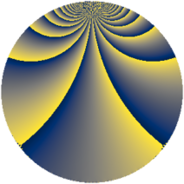# Properties

 Label 684.3.caLevel $684$ Weight $3$ Character orbit 684.ca Rep. character $\chi_{684}(193,\cdot)$ Character field $\Q(\zeta_{18})$ Dimension $240$ Sturm bound $360$

# Related objects

## Defining parameters

 Level: $$N$$ $$=$$ $$684 = 2^{2} \cdot 3^{2} \cdot 19$$ Weight: $$k$$ $$=$$ $$3$$ Character orbit: $$[\chi]$$ $$=$$ 684.ca (of order $$18$$ and degree $$6$$) Character conductor: $$\operatorname{cond}(\chi)$$ $$=$$ $$171$$ Character field: $$\Q(\zeta_{18})$$ Sturm bound: $$360$$

## Dimensions

The following table gives the dimensions of various subspaces of $$M_{3}(684, [\chi])$$.

Total New Old
Modular forms 1476 240 1236
Cusp forms 1404 240 1164
Eisenstein series 72 0 72

## Trace form

 $$240 q + 12 q^{3} - 12 q^{9} + O(q^{10})$$ $$240 q + 12 q^{3} - 12 q^{9} - 15 q^{13} - 42 q^{15} + 27 q^{17} + 21 q^{19} - 72 q^{23} - 90 q^{27} + 54 q^{29} + 102 q^{33} + 162 q^{35} - 24 q^{39} + 36 q^{41} - 48 q^{43} - 54 q^{45} - 162 q^{47} - 840 q^{49} + 9 q^{51} - 72 q^{53} - 480 q^{57} - 513 q^{59} + 21 q^{61} + 156 q^{63} + 42 q^{67} + 486 q^{71} - 111 q^{73} + 102 q^{79} - 408 q^{81} - 36 q^{83} - 144 q^{87} - 216 q^{89} + 192 q^{91} - 456 q^{93} + 216 q^{95} - 180 q^{97} + 48 q^{99} + O(q^{100})$$

## Decomposition of $$S_{3}^{\mathrm{new}}(684, [\chi])$$ into newform subspaces

The newforms in this space have not yet been added to the LMFDB.

## Decomposition of $$S_{3}^{\mathrm{old}}(684, [\chi])$$ into lower level spaces

$$S_{3}^{\mathrm{old}}(684, [\chi]) \cong$$ $$S_{3}^{\mathrm{new}}(171, [\chi])$$$$^{\oplus 3}$$$$\oplus$$$$S_{3}^{\mathrm{new}}(342, [\chi])$$$$^{\oplus 2}$$